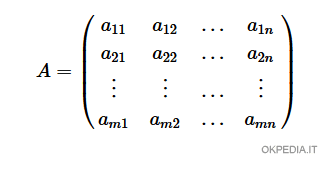# How to find the number of rows and columns of a matrix in Matlab and Octave

To find the number of rows and columns of a matrix on Matlab and Octave use the size function

size(M)

The M parameter is an array.

The function outputs the dimension of the matrix through a pair of values (m, n)

• The first element (m) is the number of rows in the matrix.
• The second element (n) is the number of columns in the matrix.Note. To output only the number of rows in the matrix, write size(M, 1). To have only the number of columns type size(M, 2).

## Examples

Example 1

Define a 3x2 rectangular matrix with two rows and three columns

$$\begin{pmatrix} 1 & 2 & 3 \\ 4 & 5 & 6 \end{pmatrix}$$

Assign the matrix to a variable

>> matrix = [ 1,2,3 ; 4,5,6 ]

To find out the size of the matrix, type the function size(matrix)

>> size(matrix)
ans = 2 3

The function returns the pair of values 2 and 3

The matrix has 2 rows and 3 columns.

Example 2

To find only the number of rows of the digital matrix size(matrix,1)

>> size(matrix,1)
ans = 2

In this case, the function outputs only the number of rows of the matrix.

Example 3

To find just the number of columns in the matrix type size(matrix,2)

>> size(matrix,2)
ans = 3

The function only outputs the number of columns in the array.

https://how.okpedia.org/en/matlab/how-to-find-the-number-of-rows-and-columns-of-a-matrix-in-matlab-and-octaveReport us an error or send a suggestion to improve this page

Matrix in Matlab/Octave